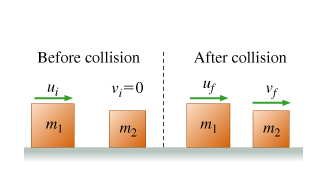# Problem: Block 1, of mass m1, moves across a frictionless surface with speed ui. It collides elastically with block 2, of mass m2, which is at rest (vi = 0). (Figure 1) After the collision, block 1 moves with speed uf, while block 2 moves with speed vf. Assume that m1 &gt; m2, so that after the collision, the two objects move off in the direction of the first object before the collision.Part AWhat is the final speed uf of block 1? Express uf in terms of m1, m2, and ui.uf = ?Part BWhat is the final speed vf of block 2? Express vf in terms of m1, m2, and ui.vf = ?

###### FREE Expert Solution

Law of conservation of linear momentum:

$\overline{){\mathbf{\sum }}{{\mathbf{P}}}_{{\mathbf{i}}}{\mathbf{=}}{\mathbf{\sum }}{{\mathbf{P}}}_{{\mathbf{f}}}}$

Part A

From the law of conservation of linear momentum, we have:

$\begin{array}{rcl}{\mathbf{m}}_{\mathbf{1}}{\mathbf{u}}_{\mathbf{i}}\mathbf{+}\mathbf{0}& \mathbf{=}& {\mathbf{m}}_{\mathbf{1}}{\mathbf{u}}_{\mathbf{f}}\mathbf{+}{\mathbf{m}}_{\mathbf{2}}{\mathbf{v}}_{\mathbf{f}}\\ {\mathbf{m}}_{\mathbf{1}}\mathbf{\left(}{\mathbf{u}}_{\mathbf{i}}\mathbf{-}{\mathbf{u}}_{\mathbf{f}}\mathbf{\right)}& \mathbf{=}& {\mathbf{m}}_{\mathbf{2}}{\mathbf{v}}_{\mathbf{f}}\end{array}$###### Problem Details

Block 1, of mass m1, moves across a frictionless surface with speed ui. It collides elastically with block 2, of mass m2, which is at rest (vi = 0). (Figure 1) After the collision, block 1 moves with speed uf, while block 2 moves with speed vf. Assume that m1 > m2, so that after the collision, the two objects move off in the direction of the first object before the collision.

Part A
What is the final speed uf of block 1? Express uf in terms of m1, m2, and ui.
uf = ?

Part B
What is the final speed vf of block 2? Express vf in terms of m1, m2, and ui.
vf = ?